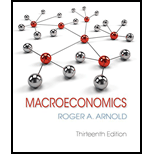# What does the nominal interest rate equal, given the following? a. Real interest rate = 3 percent; expected inflation rate = 1 percent b. Real interest rate = 5 percent; expected inflation rate = −3 percent### Macroeconomics

13th Edition
Roger A. Arnold
Publisher: Cengage Learning
ISBN: 9781337617390

#### Solutions

Chapter
Section### Macroeconomics

13th Edition
Roger A. Arnold
Publisher: Cengage Learning
ISBN: 9781337617390
Chapter 14, Problem 6WNG
Textbook Problem
1 views

## What does the nominal interest rate equal, given the following?a. Real interest rate = 3 percent; expected inflation rate = 1 percentb. Real interest rate = 5 percent; expected inflation rate = −3 percent

(a)

To determine

The nominal interest rate.

### Explanation of Solution

It is given that the real interest rate is 3 percent, and the expected inflation rate is 1 percent.

The nominal interest rate can be calculated using Equation (1) as follows:

Nominal interest rate=Real interest rate+Expected rate of inflation (1)

(b)

To determine

The nominal interest rate.

### Still sussing out bartleby?

Check out a sample textbook solution.

See a sample solution

#### The Solution to Your Study Problems

Bartleby provides explanations to thousands of textbook problems written by our experts, many with advanced degrees!

Get Started

Find more solutions based on key concepts
Explain why economists usually oppose controls prices.

Principles of Economics (MindTap Course List)

EXPECTED RETURNS Stocks X and Y have the following probability distributions of expected future returns: Probab...

Fundamentals of Financial Management, Concise Edition (with Thomson ONE - Business School Edition, 1 term (6 months) Printed Access Card) (MindTap Course List)

What is the customary date for reversing entries?

College Accounting, Chapters 1-27